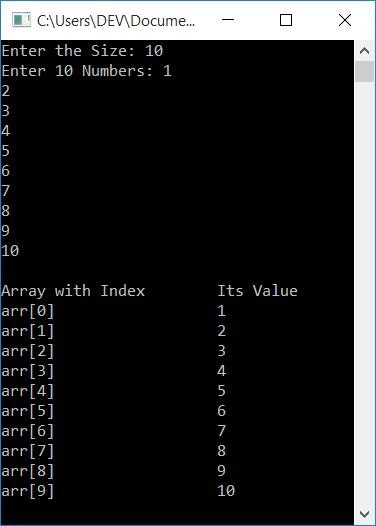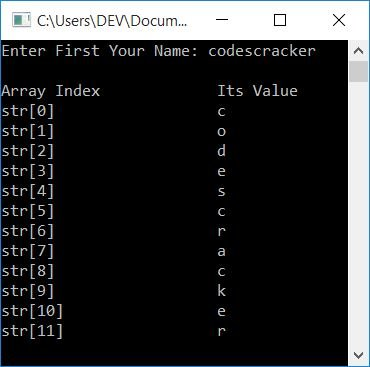# C++ program on a one-dimensional array

In this article, you will learn about and get code for a one-dimensional (1D) array in C++. For example:

`int arr = {10, 20, 30, 40, 50};`

An array variable is a variable that can hold multiple values of the same type. The term "same type" refers to the variable's (the array's variable) declared type. For example, in the above array, arr can hold up to 5 integer values.

From the above declaration, all the 5 integer values are stored in arr[] in the following way:

• arr=10
• arr=20
• arr=30
• arr=40
• arr=50

Take note that in this case, 0, 1, 2, 3, and 4 are referred to as the array's index. Indexing in an array always starts with 0.

## One Dimensional Array Program in C++

To print a one-dimensional array in C++ programming, you have to ask the user to enter the size and elements of the array. Then print it back on output with all its detail, as shown in the program given below:

```#include<iostream>
using namespace std;
int main()
{
int arr, tot, i;
cout<<"Enter the Size: ";
cin>>tot;
cout<<"Enter "<<tot<<" Numbers: ";
for(i=0; i<tot; i++)
cin>>arr[i];
cout<<"\nArray with Index\tIts Value\n";
for(i=0; i<tot; i++)
cout<<"arr["<<i<<"]"<<"\t\t\t"<<arr[i]<<endl;
cout<<endl;
return 0;
}```

This program was built and runs under the Code::Blocks IDE. Here is its sample run:Now supply inputs with 10 as the size and 1, 2, 3, 4, 5, 6, 7, 8, 9, 10 as the elements or numbers to store. Here is the sample run with exactly these inputs:The following statement appears in the preceding program:

`cout<<"arr["<<i<<"]"<<"\t\t\t"<<arr[i]<<endl;`

If we separate the items in the preceding statement into static and dynamic output (print), we get the following:

• arr[: static output. Prints the same
• i: dynamic output. Whatever the value of i is ,it is printed.
• \t\t\t: stands for static output. The three tabs are inserted on the output console.
• arr[i]: dynamic output. The value of arr[i] is printed.
• endl: static output, used as newline. That is, the next thing begins with a newline.

Note: Here, "dynamic output" means that the output gets changed with a change in the values of i and arr[i]. Things inside "" are considered static output.

The previous array is of type int (integer). Therefore, that array can hold up to 50 integer values with the same variable variable arr[], just by changing its index. Now let's try an array with its type set to char (character) to store characters.

```#include<iostream>
using namespace std;
int main()
{
char str;
int i=0;
cin>>str;
cout<<"\nArray Index\t\tIts Value\n";
while(str[i])
{
cout<<"str["<<i<<"]"<<"\t\t\t"<<str[i]<<endl;
i++;
}
cout<<endl;
return 0;
}```

Here's a test run with user input, codescracker:#### The same program in different languages

C++ Quiz

« Previous Program Next Program »